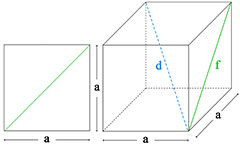# Square and Cube Calculator

The instructions for using the online Square and Cube Calculator are fairly simple. Only one known dimension of a square or cube needs to be inputted in order to find the associated values.

For squares, the user is asked to input one of the following dimensions: side length, diagonal, or area (measured in square units).

For cubes, the user is asked to input one of the following dimensions: side length, cube diagonal, face diagonal, surface area, or volume.

Square and Cube Calculator## Formulas

This calculator uses the following formulas:

Diagonal of a square = √2 × Side Length

Area of a square = Side Length 2

Diagonal of a cube = √3 × Side Length

Face Diagonal of a cube = √2 × Side Length

Surface area of a cube = 6 × Side Length 2

Volume of a cube = Side Length 3